## Train speed formula

Don't know how to use a machine? Looking for efficient setups? Stuck in a mission?
mrvn
Smart Inserter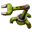Posts: 3955
Joined: Mon Sep 05, 2016 9:10 am
Contact:

### Train speed formula

I'm looking for the formula how factorio calculates train speeds. The wiki has this formula:

https://wiki.factorio.com/Locomotive#Maximum_speed
Maximum speed

The maximum speed that a locomotive can get to depends on the train that it is pulling. The speed of an accelerating train can be calculated with the following formula:

train_speed = (old_train_speed - (train_friction_force ÷ train_weight) + ((10 × number_of_locomotives_in_moving_direction × fuel_acceleration_bonus) ÷ train_weight)) × (1 - air_resistance_of_front_rolling_stock × 1000 ÷ train_weight)

where train_friction_force is amount of wagons and locomotives ÷ 2 and train_weight is the summed up weight of each wagon and locomotive, see their individual pages for the weight values. The calculated train_speed is capped to max_speed = 1.2 * fuel_top_speed_multiplier.
So every tick the train looses some speed to friction and then it gains some speed from the locmotives. Both of those factors do not include the speed of the train. So every tick the train should gain the same amount of speed until it hits the max speed. Also if the train is too long the formula turns negative, the train should loose speed every tick.

But observation show:
- A train with 1 locomotive and 128 cargo wagons moves. Terribly sow but it moves.
- A train with 1 locomotive and 32 cargo wagons accelerates from 0 at about 1km/hs. At 60km/h it accelerates about 0.5km/hs. At 80km/h it accelerates about 0.3km/hs. At 98km/h it accelerates about 0.03km/hs.

This leads me to believe that the formula for train speed in the wiki is outdated or incomplete. There clearly is a component that is dependent on the speed of the train.

Could any devs shed some light on this please?

Frightning
Filter Inserter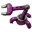Posts: 806
Joined: Fri Apr 29, 2016 5:27 pm
Contact:

### Re: Train speed formula

I agree that it would be nice for the devs to give the proper formulae for trains now, I've tried doing the math with the new vehicle modifiers on fuel and haven't been able to get numbers that match in game behavior, so the models I was using (which worked up to 0.15 more or less) is no longer valid, and in particular, don't explain how fuels w/ modifiers work.

mrvn
Smart InserterPosts: 3955
Joined: Mon Sep 05, 2016 9:10 am
Contact:

### Re: Train speed formula

Note: My LC128 train is still moving at a shown 0.0km/h at 10x speed since I started the first post. Looks like max speed for LC128 is < 0.05km/h.

FuryoftheStars
Filter InserterPosts: 389
Joined: Tue Apr 25, 2017 2:01 pm
Contact:

### Re: Train speed formula

I would really like to know this as well.

kitters
Burner Inserter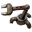Posts: 9
Joined: Tue Jul 23, 2019 4:48 pm
Contact:

### Re: Train speed formula

Look how it works, guy. Friction acts before moving power of locomotives and it can't set the speed negative, so it sets speed to zero. Locomotives act next and every tick the speed of train is equal V=[10*L*f.a./W]*[1-air/W]. If friction is stronger than moving power, a train can't accelerate and reach max speed (depending only on fuel) and moves with this min speed (depending on weight as well). If power is even a little more, even a heavy train reach the same max speed, but it would take a lot of time.

mmmPI
Filter InserterPosts: 378
Joined: Mon Jun 20, 2016 6:10 pm
Contact:

### Re: Train speed formula

kitters wrote:
Mon Aug 19, 2019 12:00 pm
Look how it works, guy. Friction acts before moving power of locomotives and it can't set the speed negative, so it sets speed to zero. Locomotives act next and every tick the speed of train is equal V=[10*L*f.a./W]*[1-air/W]. If friction is stronger than moving power, a train can't accelerate and reach max speed (depending only on fuel) and moves with this min speed (depending on weight as well). If power is even a little more, even a heavy train reach the same max speed, but it would take a lot of time.

How would you guess/know that from the wiki is beyond me , so is trying to find in the game file the part of code that would have told me this information. So thanks for that insight that would explain the general behavior of trains, i would be curious to see if tests are accurate taking this into account, but not enough to test it myself with 128 wagons trains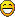Filter InserterPosts: 443
Joined: Sat May 24, 2014 7:00 am
Contact:

### Re: Train speed formula

mmmPI wrote:
Mon Aug 19, 2019 11:25 pm
How would you guess/know that from the wiki is beyond me , so is trying to find in the game file the part of code that would have told me this information. So thanks for that insight that would explain the general behavior of trains, i would be curious to see if tests are accurate taking this into account, but not enough to test it myself with 128 wagons trainsI can confirm this is approximately how it works with cars, as well. But there are some strange quirks. Like the driving power seems to be able to overcome more friction if the car (set to tank-move driving) is turning before it starts trying to move. So it's not completely clear to me how friction is computing, or why it would depend on turning, or if the turning is increasing the driving power, but generally speaking, I can confirm this is the order of equations that I've induced from my tests.

BlueTemplar
Smart InserterPosts: 2057
Joined: Fri Jun 08, 2018 2:16 pm
Contact:

### Re: Train speed formula

Hmm, vector optimizations failing on corner cases ?

Filter InserterPosts: 443
Joined: Sat May 24, 2014 7:00 am
Contact:

### Re: Train speed formula

BlueTemplar wrote:
Tue Aug 20, 2019 3:07 pm
Hmm, vector optimizations failing on corner cases ?
Yeah, possibly.

mrvn
Smart InserterPosts: 3955
Joined: Mon Sep 05, 2016 9:10 am
Contact:

### Re: Train speed formula

kitters wrote:
Mon Aug 19, 2019 12:00 pm
Look how it works, guy. Friction acts before moving power of locomotives and it can't set the speed negative, so it sets speed to zero. Locomotives act next and every tick the speed of train is equal V=[10*L*f.a./W]*[1-air/W]. If friction is stronger than moving power, a train can't accelerate and reach max speed (depending only on fuel) and moves with this min speed (depending on weight as well). If power is even a little more, even a heavy train reach the same max speed, but it would take a lot of time.
A) That's not how the formula in the wiki is written. It's a product of two parts. Not a sum of multiple parts that can be done step by step.
B) That doesn't explain the non-linear factor.

mmmPI
Filter InserterPosts: 378
Joined: Mon Jun 20, 2016 6:10 pm
Contact:

### Re: Train speed formula

quale wrote:
Wed Aug 21, 2019 4:21 pm
This made me curious. I had a bunch of train motion equations and found they no longer apply to 0.17. The cause appears to be the order of train physics. In 0.16 it was friction, drag, propulsion. That order happens to make trains the fastest, even slightly faster than idealized continuous-time differential equations describe. In 0.17 it’s friction, propulsion, drag. That’s instead slightly slower than the continuous equations. I have no idea why they changed it. Maybe it was a side effect of eliminating idle burner ticks somehow.

Top speed for various update orders (not accounting for idle ticks because that would set your brain on fire):

Continuous: v=max((p-f)/d,0)
Friction, drag, propulsion (0.16): v=max((p-f)/d+f/m,p/m)
Friction, propulsion, drag (0.17): v=max((p-f)/d-(p-f)/m,(p/m)(1-d/m))
Propulsion, friction, drag: v=max((p-f)/d-(p-f)/m,0)
Propulsion, drag, friction: v=max((p-f)/d-p/m,0)
Drag, propulsion, friction: v=max((p-f)/d,0)
Drag, friction, propulsion: v=max((p-f)/d,p/m)

v = top speed in meter/tick (multiply by 216 for km/h; ignores speed cap)
p = motive force in mass*meter/tick^2 (wood = coal = 10; solid fuel = 12; rocket fuel = 18; nuclear fuel = 25)
f = friction force in mass*meter/tick^2 (0.5 per car)
d = drag of leading car in mass/tick (7.5 for locomotive; 10 for cargo/fluid wagon; 15 for artillery wagon)
m = mass (1000 per wagon; 2000 per locomotive; 4000 per artillery wagon)

The idealized top speed doesn’t depend on mass at all; more mass just makes acceleration take longer. All deviations from ideal are inversely proportional to mass. So in 0.16 where the deviation made trains faster, more mass resulted in less speed (but always faster than continuous). In 0.17 where the deviation makes trains slower, more mass results in more speed (but always slower than continuous).

Nothing makes all that much sense in terms of real physics. But there is one update order corresponding to the most sensible math: drag, propulsion, friction. It applies drag first, to the actual speed, just like the continuous differential equation, so it converges on the same top speed. An overloaded train also doesn’t crawl (with a unit of acceleration describing speed, violating dimensional analysis) because friction is applied after propulsion. If you want to preserve crawling (I can see players would think an unmoving train spewing smoke is glitched rather than overburdened) and the little jumpstart where the first tick of acceleration is unusually strong, use drag, friction, propulsion.
This already melt my brain a little everytime i wonder for too long about it.

But, it seems to confirm the sum of multiple parts done step by step. ( and their order been changing in last updates ).

About the non-linear part one can feel free to explain to me, i'm not completly ignorant, but let's pretend i am when you explain so there is more chance i understand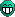quale
Inserter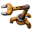Posts: 20
Joined: Thu Aug 15, 2019 4:16 pm
Contact:

### Re: Train speed formula

The wiki is correct. Note that it’s not just a sum of terms, but the speed is also multiplied by 1-drag/mass. Equivalently, you could subtract speed*drag/mass from speed. That makes drag proportional to speed. The wiki only neglects to mention the fact that the intermediate speed after friction is applied is already prevented from passing zero. Then propulsion gets to set a minimal positive speed no matter how weak it is compared to friction’s potential. kitters is right about that.
mmmPI wrote:
Thu Aug 22, 2019 5:28 pm
About the non-linear part one can feel free to explain to me, i'm not completly ignorant, but let's pretend i am when you explain so there is more chance i understandSpeed doesn’t increase at a constant rate. For example, a 1+4 coal train hits about 107.9 km/h after 554 ticks. If you wait another 554 ticks, it’ll be going less than 215.8 km/h. Only about 161.8 km/h, in fact. Another 554 ticks and it’s hit about 188.8 km/h, an even smaller gain. Waiting 554 ticks approximately halves the difference between the current speed and the top speed of 215.73 km/h for this train. The culprit is drag, which provides more resistance the faster you go.

Fun fact: Braking trains apply only the braking force. Braking from 216 km/h takes exactly twice as long as braking from 108 km/h. In fact, because the other forces are gone, it’s possible for braking to slow the train less than coasting. A lone nuclear locomotive traveling at top speed loses ~1.17 km/h on the first coasting tick. Braking would only make it lose 1.08 km/h without upgraded braking force. Such brakes start to be better under 273.654 km/h. Fully upgraded brakes manage 2.16 km/h. If you really want that same locomotive to brake hard, rotate it while continuing to press the accelerate key. Its propulsion is 25% stronger than upgraded brakes, and friction and drag do apply now. That makes it lose ~3.86 km/h on the first tick! Sadly, this is limited to lone locomotives because only those can be rotated. If it weren’t, brakes would still tend to do better in complete trains because all wagons have them.

Fun fact 2: Factorio’s trains behave much like rockets in that propulsion provides a constant force with constant fuel burn regardless of speed. They’re more efficient at higher speeds according to the Oberth effect. It’s only natural that they can run on rocket fuel.

Bilka
Factorio Staff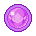Posts: 2368
Joined: Sat Aug 13, 2016 9:20 am
Contact:

### Re: Train speed formula

The wiki was slightly wrong and unreadable. It took me a bit to go through the code again but I have updated the documentation:
The maximum speed that a locomotive can get to depends on the train that it is pulling. The speed of an accelerating train can be calculated with the following formula:

Code: Select all

`````` train_speed = max(0, abs(train_speed) - train_friction_force ÷ train_weight)
train_speed = train_speed + (10 × number_of_locomotives_in_moving_direction × fuel_acceleration_bonus ÷ train_weight)
train_speed = train_speed × (1 - air_resistance_of_front_rolling_stock ÷ (train_weight ÷ 1000))``````
Where train_friction_force is the summed up friction of each wagon and locomotive and train_weight is the summed up weight of each wagon and locomotive, see their individual pages for the weight values. The calculated train_speed is capped to max_speed = 1.2 * fuel_top_speed_multiplier.
Notable changes: Slowdown by friction can at most set speed to 0, as noted above. train_friction_force was incorrect.

Notes on how to get to the formula from the source code available on request.
I'm an admin over at https://wiki.factorio.com. Feel free to contact me if there's anything wrong (or right) with it.

mrvn
Smart InserterPosts: 3955
Joined: Mon Sep 05, 2016 9:10 am
Contact:

### Re: Train speed formula

Bilka wrote:
Mon Oct 21, 2019 9:46 am
The wiki was slightly wrong and unreadable. It took me a bit to go through the code again but I have updated the documentation:
The maximum speed that a locomotive can get to depends on the train that it is pulling. The speed of an accelerating train can be calculated with the following formula:

Code: Select all

`````` train_speed = max(0, abs(train_speed) - train_friction_force ÷ train_weight)
train_speed = train_speed + (10 × number_of_locomotives_in_moving_direction × fuel_acceleration_bonus ÷ train_weight)
train_speed = train_speed × (1 - air_resistance_of_front_rolling_stock ÷ (train_weight ÷ 1000))``````
Where train_friction_force is the summed up friction of each wagon and locomotive and train_weight is the summed up weight of each wagon and locomotive, see their individual pages for the weight values. The calculated train_speed is capped to max_speed = 1.2 * fuel_top_speed_multiplier.
Notable changes: Slowdown by friction can at most set speed to 0, as noted above. train_friction_force was incorrect.

Notes on how to get to the formula from the source code available on request.
Isn't it "10 * SUM(locomotive_in_moving_direction × fuel_acceleration_bonus_of locomotive)"? Each locomotive can have a different fuel.

Bilka
Factorio StaffPosts: 2368
Joined: Sat Aug 13, 2016 9:20 am
Contact:

### Re: Train speed formula

mrvn wrote:
Mon Oct 21, 2019 10:35 am
Isn't it "10 * SUM(locomotive_in_moving_direction × fuel_acceleration_bonus_of locomotive)"? Each locomotive can have a different fuel.
Yes, the wiki indeed assumes same fuel for all locomotives. Same goes for the top speed cap. Assuming different fuel (and possibly non-vanilla locos and different locos in the train) would complicate the formula a lot so it is not done on the wiki, however the game itself respects all of that.
I'm an admin over at https://wiki.factorio.com. Feel free to contact me if there's anything wrong (or right) with it.

Cribbit
Fast Inserter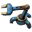Posts: 193
Joined: Mon Oct 09, 2017 9:35 pm
Contact:

### Re: Train speed formula

So https://calculatorio.com/train_acceleration/ is accurate then?

I've been thinking of forking that and adding some graphs around throughput, speed curve, time to travel X distance etc

I had been putting it off but https://www.reddit.com/r/factorio/comme ... _or_how_i/ gave me fresh inspiration!

Bilka
Factorio StaffPosts: 2368
Joined: Sat Aug 13, 2016 9:20 am
Contact:

### Re: Train speed formula

Cribbit wrote:
Mon Oct 21, 2019 12:44 pm
So https://calculatorio.com/train_acceleration/ is accurate then?
No, it's using the old code that was on the wiki which has the wrong friction calc.

I'm an admin over at https://wiki.factorio.com. Feel free to contact me if there's anything wrong (or right) with it.

Cribbit
Fast InserterPosts: 193
Joined: Mon Oct 09, 2017 9:35 pm
Contact:

### Re: Train speed formula

Bilka wrote:
Mon Oct 21, 2019 1:08 pm
Cribbit wrote:
Mon Oct 21, 2019 12:44 pm
So https://calculatorio.com/train_acceleration/ is accurate then?
No, it's using the old code that was on the wiki which has the wrong friction calc.